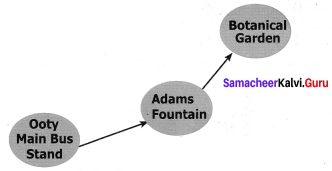Students can Download Maths Chapter 6 Information Processing Intext Questions and Answers, Notes Pdf, Samacheer Kalvi 7th Maths Book Solutions Guide Pdf helps you to revise the complete Tamilnadu State Board New Syllabus and score more marks in your examinations.

## Tamilnadu Samacheer Kalvi 7th Maths Solutions Term 1 Chapter 6 Information Processing Intext Questions

Exercise 6.1

Try this (Text book Page No. 111)

Question 1.
Use the given five tetrominoes only once and create the shape given below.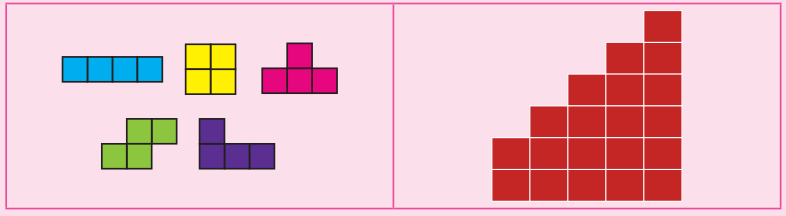Solution:
Using the given five tetrominoes in the proper places we can make the given shape as follows.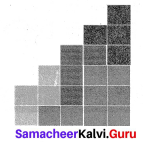Try these (Text Book Page No. 113)

Question 1.
Complete the rectangle given below using five tetrominoes only once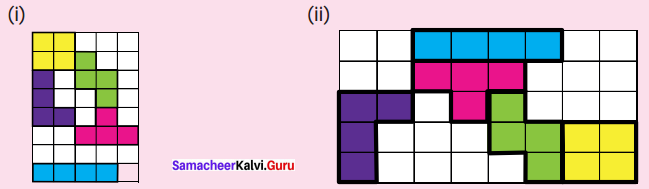Solution:
The given rectangle is halfly filled with the five tetrominoes.
Using the five tetrominoes only once we can fill the rectangles as follows: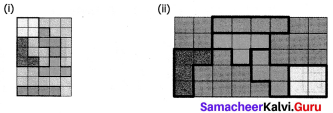Question 2.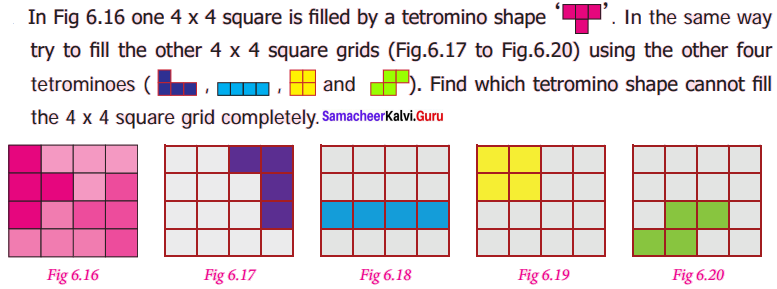Solution: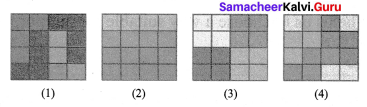Exercise 6.2

Try these (Text Book Page No. 119)

Question 1.
Observe the pictures and answer the following.
(i) Find all the possible routes from house to school via fire station.
(ii) Find all the possible routes between central park and school with distance. Mention the shortest route?
(iii) Calculate the shortest distance between bank and school.
Solution:
(i) (a) House ➝ Fire station ➝ Library ➝ Central Park ➝ Hotel ➝ Fruit shop ➝ School.
(b) House ➝ Fire station ➝ Library ➝ Fruit shop ➝ School.
(c) House ➝ Fire station ➝ Library ➝ School.

(ii) Possible routes between Central park and school and their distances are
(a) School ➝ Fruit shop ➝ Hotel ➝ Central park.
Distance ➝ (150 + 300 + 100)m = 550 m

(b) School ➝ Fruit shop ➝ Library ➝ Central park Distance ➝(150 + 100 + 200)m
= 450 m

(c) School ➝ Library ➝ Central park
Distance = (20 + 200 m)
= 220 m

(d) School ➝ Library ➝ Fire station ➝ House ➝ central park Distance = (20 + 50 + 300 + 150) m
= 520 m

(e) School ➝ Emit shop ➝ Hotel ➝ Bank ➝ House ➝ Central park
Distance ➝ (150 + 300 + 150 + 200 + 150) m
= 950 m

(f) School ➝ fruit shop ➝ Hotel ➝ Bank ➝ House ➝ Fire station ➝ Library ➝ central park
Distance = (150 + 300 + 150 + 200 + 300 + 50 + 200)m = 1350 m
∴ Route (c) is the shortest path (i.e.,) School ➝ Library ➝ Central park

(iii) Shortest distance between school and Bank is calculated as follows Bank ➝ Hotel ➝ Central park ➝ Library ➝ school.
Distance = (150 + 100 + 200 + 20)m
= 470 m

Question 2.
A School has planned for a trip to Ooty. Using the route map, the school decides to visit the places such as Boat House Adam Fountain and Botanical Garden.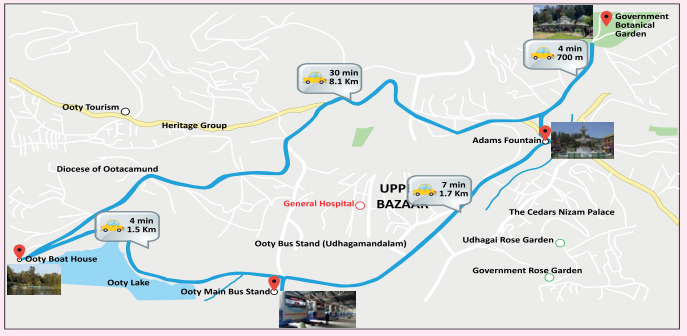(i) How much distance you have to travel to Botanical Garden from Ooty Boat House?
(ii) Find the shortest route to Botanical from the Ooty main Bus stand.
(iii) Mention the direction of Botanical Garden from Adam Foundation.
(iv) In what direction, Ooty Boat House is situated from Ooty Main Bus Stand. Complete the following route map from Ooty Main Bus Stand to Botanical Garden.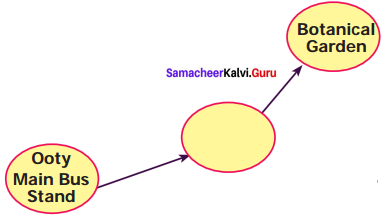Solution:
(i) (a) 700 m + 8.1 km = 700 m + (8 km + 100 m) = 8 km + 800 m = 8.8 km
(b) 700 m+ 1.7 km + 1.5 km = 700 m + (1 km, 700 m) + 1 km 500 m
= 2 km + 1900 m
= 2 km + 1 km + 900 m
= 3 km + 900 m
= 3.9 km
From the Ooty Boat House, the Botanical Garden is at a distance of 3.9 km(shortest)

(ii) The route from Botanical Garden from Ooty main Bus stand are
(a) Ooty main Bus stand ➝ Boat house ➝ Government Botanical garden Distance 1.5 km + 8.1 km + 700 m
= 1 km 500 m + 8 km 100 m + 700 m
= 9 km 1300 m
= 9 km + 1 km +300 m
= 10 km 300 m
= 10.3 km.

(b) Another route.
Ooty Main Bus stand ➝ Adam Fountain ➝ Botanical garden.
Distance = 1.7 + 700 m
= 1 Km 700 m + 700m
= 1 Km 1400 m
= 1 Km+ 1 Km 400 m
= 2 Km 400 m
= 2.4Km
∴ Shortest Route is Ooty main Bus stand ➝ Adams Foundation ➝ Botanical garden

iii. Botanical garden is north of Adam Foundation.

iv. Ooty Boat House is situated to the west of Ooty main bus bus stand.

v.